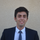cancel
Showing results for
Did you mean:

Fabric is Generally Available. Browse Fabric Presentations. Work towards your Fabric certification with the Cloud Skills Challenge.Frequent Visitor

## DAX query for Average

Hello,

I need to calculate the average sale using the formula: [ProductA amount for a month] / [ProductB amount for a month]

For example, for the Month of January 2016, ProductA amount = 1000 and ProductB amount = 10

for the Month of January 2017, ProductA amount = 2000 and ProductB amount = 20

Then if I select January as month and 2016 and 2017 as Year, average should be (1000 + 2000)/(10+20)

Consider the scenaio where I need to calculate the average for multiple months and one of the value in denominator is null:

for the Month of January 2016, ProductA amount = 1000 and ProductB amount = 0

for the Month of January 2017, ProductA amount = 2000 and ProductB amount = 20

Then average should be 2000/20. Need to ignore January 2016 sale amount since the denominator is 0.

Can anyone help me to resolve this.

2 REPLIES 2MVP

Well it is hard to help you without seeing the tables at stake but let's give it a try.

First, you need to have a proper Calendar Table to perform any Time Intelligence calculations.

Second, be aware that you don't need to select 2 years simultaneously. Selecting 1 year through a slicer and going 1 year backward using DAX will be good (unless you want to compare 2017 vs 2015... which would not make sense right?).

So, supposing the user always selects a single year and a single month, create the following measures:

Total Amount = Sum ( YourTable[Amount] )

AmountA = Calculate ( [Total Amount] , ProductTable[ProductType] = "A")

AmountALastY = Calculate ( [AmountA] , PreviousMonth(CalendarTable[Date] )

AmountB= Calculate ( [Total Amount] , ProductTable[ProductType] = "B")

AmountBLastY = Calculate ( [AmountB] , PreviousMonth(CalendarTable[Date] )

CustomAvg = If ( [AmountALastY] = 0 , Divide ( [AmountB] , [AmountBLastY] ) , If ([AmountBLastY] = 0, Divide ( [AmountA] , [AmountALastY] ) , Divide ( [AmountA] + [AmountB] , [AmountALastY] + [AmountBLastY]) )

You need to add another condition if both A and B were not sold last year but I am sure you got the logic 🙂Frequent Visitor

Thanks for taking time out and replying to my query.

My table structure is as follows:

Both numerator and denominator is being taken as SUM(Amount)  where Amount is a column in two different fact tables(Fact1 and Fact2). Fact1 and Fact2 doesn't have a direct relationship between them but these two fact tables have a direct relationship with a Date table. This Date table is also used in my Year and Month slicer.

The denominator is not 0 but missing value for some months in 2016. If I only select 2016, it will not show any rate since denominator is missing as expected. But if I select 2016 and 2017, it is calculating the rate as sum of two numerators(amount for 2016 and 2017) by the denominator of 2017(since 2016 denominator is missing for few months) which should not be happening. I want to ignore the 2016 numerator and calculate average of percentage across any date range.

I hope I am clear. How can I achieve this?Announcements#### Power BI Monthly Update - November 2023

Check out the November 2023 Power BI update to learn about new features.#### Fabric Community News unified experience

Read the latest Fabric Community announcements, including updates on Power BI, Synapse, Data Factory and Data Activator.#### The largest Power BI and Fabric virtual conference

130+ sessions, 130+ speakers, Product managers, MVPs, and experts. All about Power BI and Fabric. Attend online or watch the recordings.Top Solution Authors
Top Kudoed Authors
Users online (2,640)